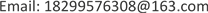﻿ 中国城市居民农产品需求结构研究——基于ELES模型食品消费需求的实证 Research on the Demand Structure of Agricultural Products of Chinese Urban Res-idents—An Empirical Study Based on the ELES Model of Food Consumption Demand

Hans Journal of Agricultural Sciences
Vol. 09  No. 05 ( 2019 ), Article ID: 30287 , 8 pages
10.12677/HJAS.2019.95052

Research on the Demand Structure of

Agricultural Products of Chinese Urban Residents

Wenyu Zhang

College of Economics and Management, Tarim University, Alaer XinjiangReceived: May 1st, 2019; accepted: May 13th, 2019; published: May 20th, 2019—An Empirical Study Based on the ELES Model of Food Consumption Demand

ABSTRACT

The demand for agri-food is the basic demand of residents. In economic and social development, the quantity, quality and volume of food demand of residents are also changing. Using the extended linear expenditure system model ELES, an empirical analysis of the marginal propensity to consume, demand income elasticity and basic demand of Chinese urban residents in 2017 shows that urban residents have a strong preference for food, vegetables, dried fruits, meat, and aquatic products. The growth of urban residents’ demand for food consumption is less than the growth of per capita disposable income, which is not sensitive to changes in income. The focus of urban residents’ food consumption is mainly on food, cooking oil and vegetables. Urban residents should pay more attention to the balanced diet and the food consumption structure needs to be further optimized.

Keywords:Agricultural Food Consumption, Extended Linear Expenditure System, Marginal Propensity to Consume, Demand Income Elasticity

——基于ELES模型食品消费需求的实证1. 引言

2. 文献综述

3. 扩展线性支出系统(ELES) 模型的构建与检验

3.1. ELES模型的构建

3.1.1. 模型理论

ELES (扩展线性支出系统)模型假定某一时期人们对各种商品(服务)的需求量取决于人们的收入和各种商品的价格，而且人们对各种商品的需求分为基本需求和超过基本需求之外的需求两部分，并且认为基本需求与收入水平无关，居民在基本需求得到满足之后才将剩余收入按照某种 边际消费倾向安排各种非基本消费支出。

${P}_{i}{X}_{i}={P}_{i}{X}_{i0}+{b}_{i}\left(Y-\sum {P}_{i}{X}_{i0}\right)\left(i=1,2,\cdots n\right)$ (1)

$令{a}_{i}={P}_{i}{X}_{i0}-{b}_{i}\sum {P}_{i}{X}_{i0}\left(i=1,2,\cdots n\right)$ (2)

${V}_{i}={P}_{i}{X}_{i}={a}_{i}+{b}_{i}Y\left(i=1,2,\cdots n\right)$ (3)

$令\sum {a}_{i}=\sum {P}_{i}{X}_{i0}-\sum {b}_{i}\sum {P}_{i}{X}_{i0},可得到\sum {P}_{i}{X}_{i0}=\sum {a}_{i}/\left(1-\sum {b}_{i}\right)$

${P}_{i}{X}_{i0}={a}_{i}+{b}_{i}\sum {P}_{i}{X}_{i0}={a}_{i}+{b}_{i}\sum {a}_{i}/\left(1-\sum {b}_{i}\right)$ (4)

${E}_{m}={b}_{i}*Y/\left({P}_{i}{X}_{i}\right)$ (5)

3.1.2. 变量的选取与所用数据。

3.2. ELES模型的参数估计Table 1. Coefficient of 2017 ELES model and demand income elasticity calculation results

$V1=489.991889388+0.0330115808932*Y$ (6)

$V2=45.4420926879+0.00330330703456*Y$ (7)

$V3=55.9389908673+0.0395653006595*Y$ (8)

$V4=2.14857662085+0.0118966567111*Y$ (9)

$V5=-62.1620240128+0.00581005307968*Y$ (10)

$V6=-302.8893769+0.0150493349465*Y$ (11)

$V7=-56.8711486507+0.00580911497442*Y$ (12)

$V8=-102.689139594+0.0102603749607*Y$ (13)

$V9=-125.832534561+0.0292465261979*Y$ (14)

$V10=1.94293180573+0.000521255765667*Y$ (15)

3.3. ELES模型检验

1) 经济意义检验。对于设定模型的参数来说，参数的估计值的经济含义是当人均可支配收入增加1元时，城市居民对食品的消费支出将增加的量。根据日常生活经验和普遍经济意义来看，无论是食品整体还是粮食、肉禽及其制品、蛋类、水产品、奶及奶制品和其它食品，人均可支配收入的增加都会带动居民对它们的需求，从而提高对它们的消费支出，故对于文中所研究的对象来说，回归模型中都有参数bi > 0。

2) 统计学检验。从回归估计的结果可以看到，在α = 0.05的显著水平下，各类消费的回归方程军通过F检验，解释变量也均通过了t检验，这表明模型的整体解释效果较好，2017年我国城市居民的人均可支配收入对食品中粮食、食用油、蔬菜及食用菌、肉类、禽类、水产品、蛋类、奶类、干鲜瓜果类、食糖的消费支出的影响都是显著的。说明我国城镇居民可支配收入对各项消费支出高度相关，消费支出方程的斜率均在0~1之间，符合模型中关于0 < bi < 1的要求。

3) 计量经济学检验。本文采用的是2017年全国各地区城镇居民家庭人均食品消费支出和可支配收入的截面数据，一方面由于各城市之间贫富差距较大，可支配收入各不相同，因此，对各种食品的消费支出会存在差异，这种差异使得模型很容易产生异方差性，从而影响模型的估计和应用；另一方面由于Y的各收入阶层居民占比各有不同，有的是按10%，有的是按20%，而由统计学可知，一个组包括的样本量越大，该组的平均值变异越小，使用这种数据建立模型时数据本身的生成过程就体现了异方差。为此必须对模型是否存在异方差进行检验。经检验模型存在异方差性，因此进行了异方差的修正。

4. 中国城市居民食品消费实证分析

4.1. 边际消费倾向分析

4.2. 需求收入弹性分析

4.3. 基本需求支出分析Table 2. The proportion of basic needs of various types of food consumption of urban residents

2017年我国城市居民在各类食品上的基本需求支出均远远小于人均可支配收入，这表明城市居民的基本食品需要得到了充分的满足。表2显示，2017年我国城镇居民基本消费支出主要集中在粮食(39.2%)、水产品(24.42%)、干鲜瓜果(10.26%)三类，合计占基本食品消费支出的73.88%。而在十类食品中，粮食在城市居民的食品基本需求支出中占据了最大的比例，达到了39.2%；这表示我国城市居民在消费这十类基础食品时，往往把重心放粮食上，需注重饮食均衡。在模型估计中的常数项可以用来判断随着收入水平的上升，该类商品的支出所占比重的变动趋势。若常数项小于0，则随着收入的增加，该类商品的支出比重将上升；反之，若常数项大于0，则随着收入的增加，该类商品的支出比重将下降。从表1数据可以看到，粮食、食用油、蔬菜及食用菌、肉类、食糖的常数项均大于0，这表示随着城市居民收入的上涨，上述五类食品的消费支出所占比重会逐渐下降，禽类、水产品、蛋类、奶类、干鲜瓜果类常数项均小于0，这表示随着城市居民收入的上涨，上述五类食品的消费支出所占比重会逐渐上升，这也是城市居民生活水平不断提高的表现。

5. 结论

Research on the Demand Structure of Agricultural Products of Chinese Urban Res-idents—An Empirical Study Based on the ELES Model of Food Consumption Demand[J]. 农业科学, 2019, 09(05): 350-357. https://doi.org/10.12677/HJAS.2019.95052

1. 1. Stone, R. (1954) Linear Expenditure System and Demand Analysis: An Application to the Pattern of British Demand. The Economic Journal, 64, 511-527. https://doi.org/10.2307/2227743

2. 2. Constantino, L. (1973) The Extended Linear Expenditure System. European Economic Review, 4, 21-23. https://doi.org/10.1016/0014-2921(73)90028-7

3. 3. Deaton, A.S. and Muellbauer, J. (1980) An Almost Ideal Demand System. American Economic Review, 70, 312-316.

4. 4. 朱高林. 中国城镇居民食品消费的新变化[J]. 经济研究参考, 2018(13): 42-48 + 79.

5. 5. 王德章, 王甲樑. 新形势下我国食品消费结构升级研究[J]. 农业经济问题, 2010(6): 75-79.

6. 6. 王震, 刘伟平, 江晓敏, 等. 基于ELES模型的福建省城镇居民食物消费需求研究[J]. 福建农林大学学报: 哲学社会科学版, 2015, 18(1): 61-65.

7. 7. 邓隽. 武汉市城镇居民食品消费结构实证分析[J]. 华中农业大学学报: 社会科学版, 2011(2): 125-129.

8. 8. 朱海玲. 湖南城镇居民食品消费ELES模型弹性分析[J]. 食品与机械, 2007, 23(3): 163-165.

9. 9. 孙赫, 任金政. 基于ELES模型的中国城镇居民食品消费结构实证分析[J]. 农业展望, 2014, 10(7): 70-74.